# = cos x sin y 5. [MT, p. 166] Calculate the second-order Taylor approximation to f(x,...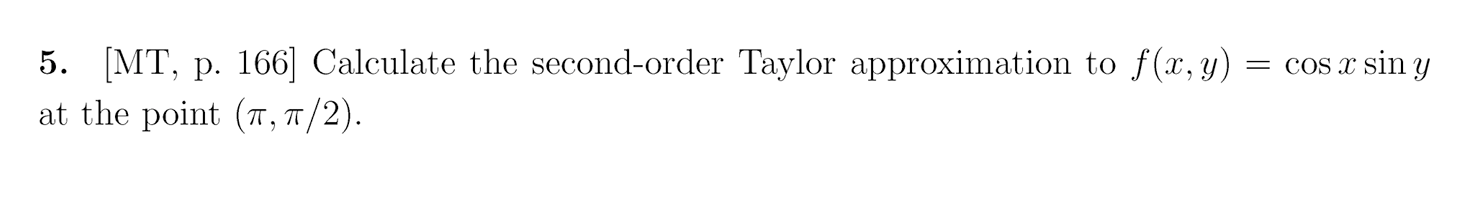= cos x sin y 5. [MT, p. 166] Calculate the second-order Taylor approximation to f(x, y) at the point (7, 7/2).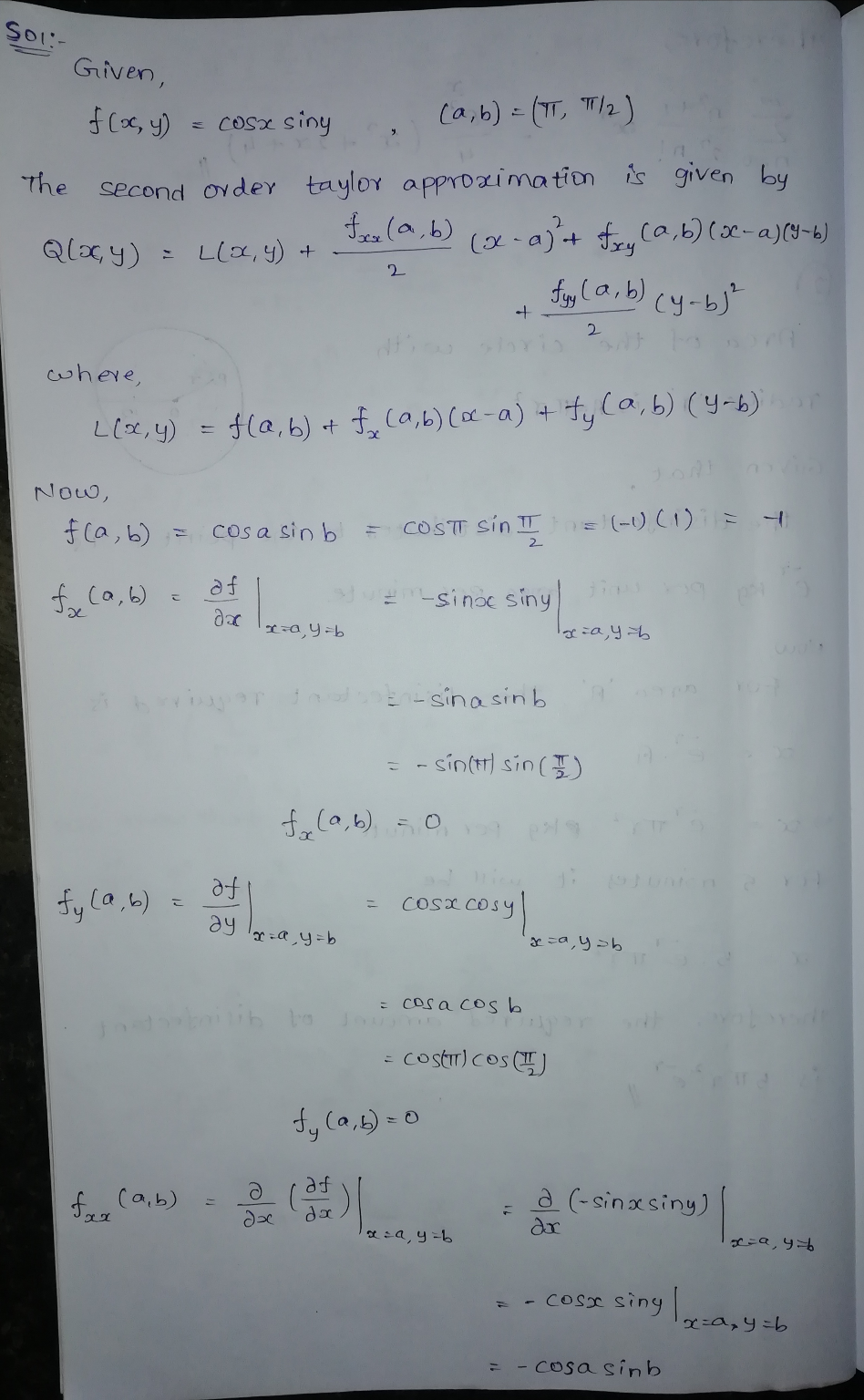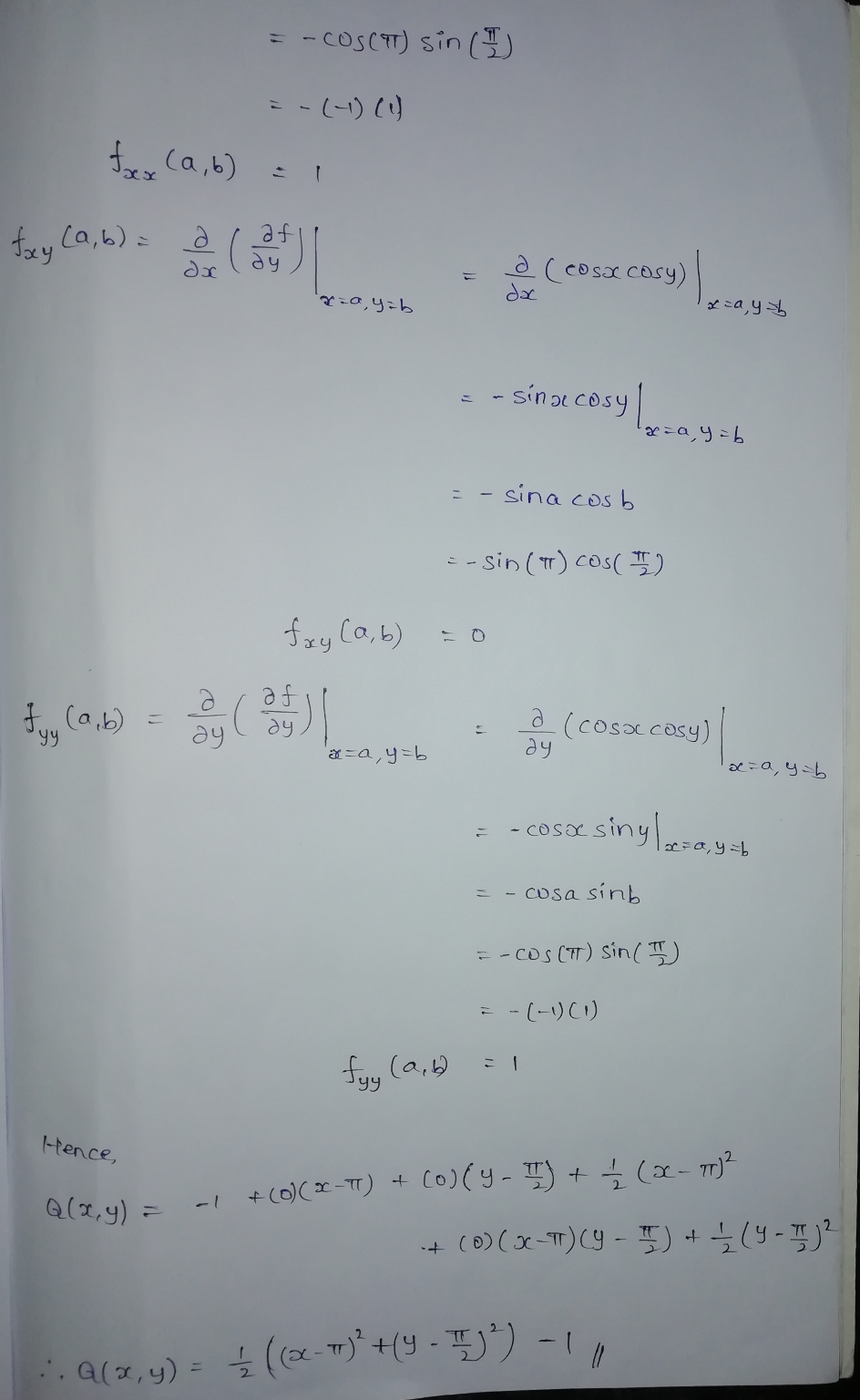If you have any dought please comment and give me a like if the answer is clear. Thank you

##### Add Answer of: = cos x sin y 5. [MT, p. 166] Calculate the second-order Taylor approximation to f(x,...
Similar Homework Help Questions
• ### 5. Find the 2nd-order Taylor approximation of f(x, y) = el+22 –y? around the critical point...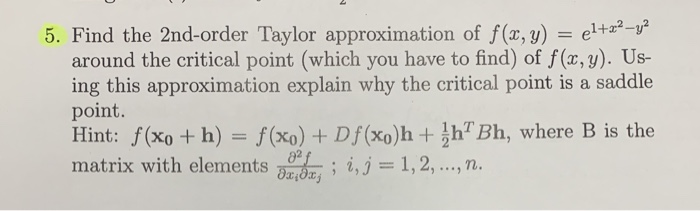5. Find the 2nd-order Taylor approximation of f(x, y) = el+22 –y? around the critical point (which you have to find) of f(x,y). Us- ing this approximation explain why the critical point is a saddle point. Hint: f(xo + h) = f(x0) + Df(xo)h + ihBh, where B is the matrix with elements on your ; i, j = 1, 2, ..., n.

• ### Exam 2018s1] Consider the function f R2 R, defined by f(x,y) =12y + 3y-2 (a) Find the first-order Taylor approximation at the point Xo-(1,-2) and use it to find an approximate value for f(1.1,-2.1 (b...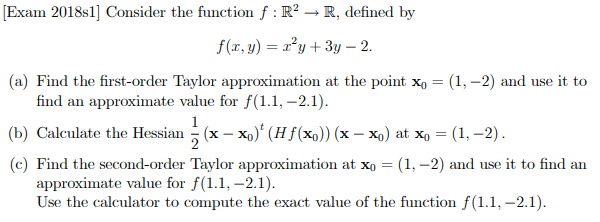Exam 2018s1] Consider the function f R2 R, defined by f(x,y) =12y + 3y-2 (a) Find the first-order Taylor approximation at the point Xo-(1,-2) and use it to find an approximate value for f(1.1,-2.1 (b) Calculate the Hessian 1 (x-4)' (Hr(%)) (x-%) at X-(1-2) c) Find the second-order Taylor approximation at xo- (1,-2) and use it to find an approximate value for f(1.1,-2.1 Use the calculator to compute the exact value of the function f(11,-2.1) Exam 2018s1] Consider the function...

• ### The Taylor polynomial approximation pn (r) for f(x) = sin(x) around x,-0 is given as follows: TL ...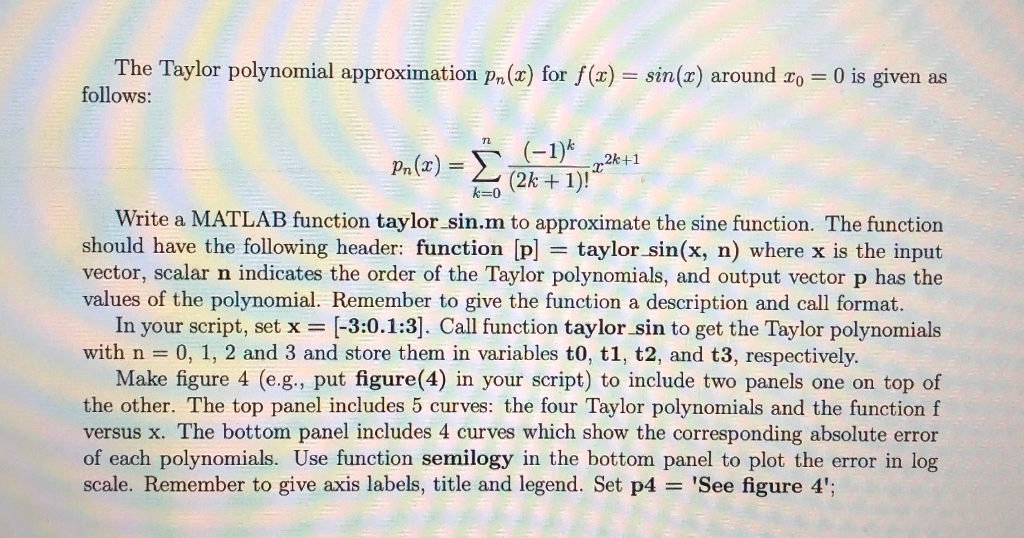The Taylor polynomial approximation pn (r) for f(x) = sin(x) around x,-0 is given as follows: TL 2k 1)! Write a MATLAB function taylor sin.m to approximate the sine function. The function should have the following header: function [p] = taylor-sin(x, n) where x is the input vector, scalar n indicates the order of the Taylor polynomials, and output vector p has the values of the polynomial. Remember to give the function a description and call format. in your script,...

• ### Tangent plane, directional dervative, second order Taylor expansion

Let f(x; y) = x cos(x) cos(y)Find the tangent plane to the surface defined by f(x; y) at thepoint (π ; π ; π ).Compute the directional derivative of f(x; y) at the point (π;π/2 ) in the direction of (i-j)/sqrt(2).Compute the second order Taylor expansion of f(x; y) about thepoint (0,0)

• ### Compute a FD second order approximation of the first derivative of the function f(x) = sin(x2) at...

Compute a FD second order approximation of the first derivative of the function f(x) = sin(x2) at x = 1.5 using x = 0.1

• ### Use a two-dimensional Taylor series to find a linear approximation for the function f (2,y) =...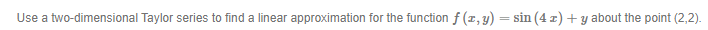Use a two-dimensional Taylor series to find a linear approximation for the function f (2,y) = sin (42) +y about the point (2,2).

• ### Find Ts(x): Taylor polynomial of degree 5 of the function f(z) -cos( at a0 Preview Using the Taylor Remainder Theorem, find all values of x for which this approximation is within 0.002412 of the righ...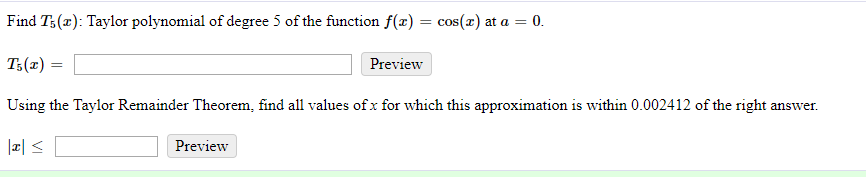Find Ts(x): Taylor polynomial of degree 5 of the function f(z) -cos( at a0 Preview Using the Taylor Remainder Theorem, find all values of x for which this approximation is within 0.002412 of the right answer Preview Find Ts(x): Taylor polynomial of degree 5 of the function f(z) -cos( at a0 Preview Using the Taylor Remainder Theorem, find all values of x for which this approximation is within 0.002412 of the right answer Preview

• ### For the force field F(x, y, z) = (zex cos y) i - (zex sin y) j + (ex cos y) k, calculate the...

For the force field F(x, y, z) = (zex cos y) i - (zex sin y) j + (ex cos y) k, calculate the work done by F on an object moving along a curve from (0, 0, 2) to (1,p/2, 3) .

• ### 8. Determine the first three nonzero terms in the Taylor approximation for y" + cos y...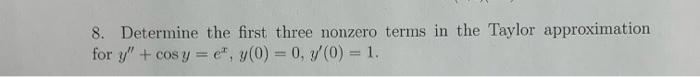8. Determine the first three nonzero terms in the Taylor approximation for y" + cos y = e", y(0) = 0, 7(0) = 1.

• ### Second-Order Taylor Formula

Determine the second-order Taylor formula for the given function about the given point (xo,yo).f(x,y) = (e^((x-1)^2))(cosy) , where xo=1, yo=0

Free Homework App